Some equipotential surfaces of the magnetic scalar potential are shown in the figure. The magnetic field at a point in the region is (X-axis read in cm)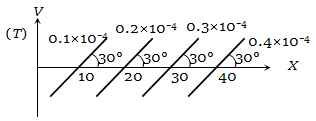(a)                         (b)

(c)                  (d) None of these

Concept Questions :-

Analogy between electrostatics and magnetostatics
High Yielding Test Series + Question Bank - NEET 2020

Difficulty Level:

The figure illustrates how B, the flux density, inside a sample of unmagnetized ferromagnetic material varies with B0, the magnetic flux density, in which the sample is kept. For the sample to be suitable for making a permanent magnet -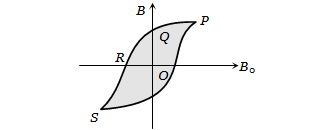1. OQ should be large and OR should be small

2. OQ and OR should both be large

3. OQ should be small and OR should be large

4. OQ and OR should both be small

Concept Questions :-

Curie law and hyterisis
High Yielding Test Series + Question Bank - NEET 2020

Difficulty Level:

The variation of the intensity of magnetisation (I) with respect to the magnetising field (H) in a diamagnetic substance is described by the graph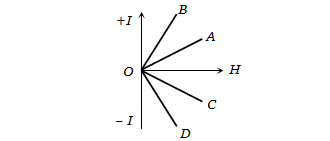1. OD                            2. OC

3. OB                            4. OA

Concept Questions :-

Magnetization and magnetic intensity
High Yielding Test Series + Question Bank - NEET 2020

Difficulty Level:

If  be the apparent angles of dip observed in two vertical planes at right angles to each other, then the true angle of dip $\theta$ is given by

(a) $co{t}^{2}\theta =co{t}^{2}{\theta }_{1}+co{t}^{2}{\theta }_{2}$

(b) ${\mathrm{tan}}^{2}\theta ={\mathrm{tan}}^{2}{\theta }_{1}-{\mathrm{tan}}^{2}{\theta }_{2}$

(c) $co{t}^{2}\theta =co{t}^{2}{\theta }_{1}-{\mathrm{tan}}^{2}{\theta }_{2}$

(d) ${\mathrm{tan}}^{2}\theta ={\mathrm{tan}}^{2}{\theta }_{1}-{\mathrm{tan}}^{2}{\theta }_{2}$

Concept Questions :-

Earth's magnetism
High Yielding Test Series + Question Bank - NEET 2020

Difficulty Level:

A bar magnet is hung by a thin cotton thread in a uniform horizontal magnetic field and is in the equilibrium state. The energy required to rotate it by ${60}^{\circ }$ is W. Now the torque required to keep the magnet in this new position is

1. $\frac{W}{\sqrt{3}}$                    2. $\sqrt{3}W$

3. $\frac{\sqrt{3}W}{2}$                4. $\frac{2W}{\sqrt{3}}$

Concept Questions :-

Analogy between electrostatics and magnetostatics
High Yielding Test Series + Question Bank - NEET 2020

Difficulty Level:

The magnetic susceptibility is negative for

1. paramagnetic material only

2. ferromagnetic material only

3. paramagnetic and ferromagnetic materials

4. diamagnetic material only

Concept Questions :-

Magnetic materials
High Yielding Test Series + Question Bank - NEET 2020

Difficulty Level:

The following figures show the arrangement of bar magnet in different configurations. Each magnet has magnetic dipole moment m. Which configuration has the highest net magnetic dipole moment?
(i)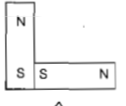(ii)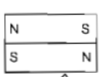(iii)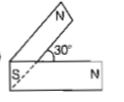(iv)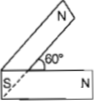1. (i)

2. (ii)

3. (iii)

4. (iv)

Concept Questions :-

Bar magnet
High Yielding Test Series + Question Bank - NEET 2020

Difficulty Level:

A compass needle which is allowed to move in a horizontal plane is taken to a geomagnetic pole.It

(a) will become rigid showing no movement

(b) will stay in any position

(c) will stay in north-south direction only

(d) will stay in east-west direction only

Concept Questions :-

Magnetic field and field lines
High Yielding Test Series + Question Bank - NEET 2020

Difficulty Level:

A magnetic needle suspended parallel to a

magnetic field requires $\sqrt{3}$ J of work to turn

it through $60°$. The torque needed to maintain

the needle in this position will be

(a) $2\sqrt{3}$J                      (b) 3 J

(c) $\sqrt{3}$ J                       (d) $\frac{3}{2}$ J

Concept Questions :-

Bar magnet
High Yielding Test Series + Question Bank - NEET 2020

Difficulty Level:

There are four light-weight-rod samples; A, B, C, D separately suspended by threads. A bar magnet is slowly brought near each sample and the following observations are noted:

(i) A is feebly repelled

(ii) B is feebly attracted

(iii) C is strongly attracted

(iv) D remains unaffected

Which one of the following is true?

1. C is of a diamagnetic material

2. D is of a ferromagnetic material

3. A is of a non-magnetic material

4. B is of a paramagnetic material

Concept Questions :-

Magnetic materials